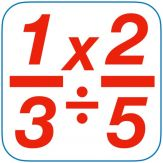Español

Cada día ofrecemos un programa licenciado GRATIS que de otra manera tendrías que comprar!\$1.99
EXPIRÓ

# iPhone Giveaway of the Day - TiM Fractions 2

A total of 7 quizzes, each quiz builds on skills learned in previous quizzes.
\$1.99 EXPIRÓ

Esta oferta de regalo ha expirado. TiM Fractions 2 ya está disponible.

Features:
1. A total of 7 quizzes, each quiz builds on skills learned in previous quizzes.
2. 12 instructional videos, 1 or more for each qiuiz.
3. Records quiz results for up to 10 learners (locally only) on device being used.
4. High-quality feedback for incorrect answers, matched to each problem.
5. Help screens available for each problem in each quiz.

Content by quiz:

Quiz 1 – Fraction multiplication, simplifying not required.

Quiz 2 – Fractions times whole numbers with fraction products, simplifying fractions not required.

Quiz 3 – Fractions times whole numbers with mixed number products, simplifying fractions not required.

Quiz 4 – Mixed number multiplication with mixed number product, simplifying fraction not required.

Quiz 5 – Mixed number multiplication, simplifying required (only 1 GCF involved).

Quiz 6 – Mixed number multiplication, simplifying required (2 GCFs involved).

Quiz 7 – Fraction and mixed number division, simplifying required.

David Mcclintock

Education

1.1

479.79 MB

4+

### Idiomas:

English